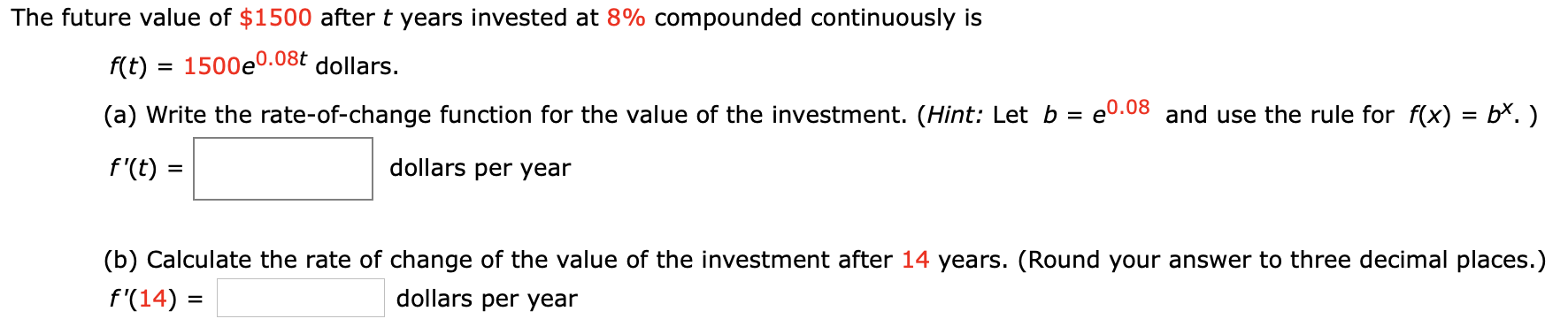# The future value of \$1500 after t years invested at 8% compounded continuously isf(t)1500e0.08t dollars.e0.08 and use the rule for f(x) = b*.)(a) Write the rate-of-change function for the value of the investment. (Hint: Let bdollars per yearf'(t)=(b) Calculate the rate of change of the value of the investment after 14 years. (Round your answer to three decimal places.)dollars per yearf'(14)

Question
1 viewshelp_outlineImage TranscriptioncloseThe future value of \$1500 after t years invested at 8% compounded continuously is f(t)1500e0.08t dollars. e0.08 and use the rule for f(x) = b*.) (a) Write the rate-of-change function for the value of the investment. (Hint: Let b dollars per year f'(t) = (b) Calculate the rate of change of the value of the investment after 14 years. (Round your answer to three decimal places.) dollars per year f'(14) fullscreen
check_circle

Step 1

a) the rate of change for the va...

### Want to see the full answer?

See Solution

#### Want to see this answer and more?

Solutions are written by subject experts who are available 24/7. Questions are typically answered within 1 hour.*

See Solution
*Response times may vary by subject and question.
Tagged in

### Other